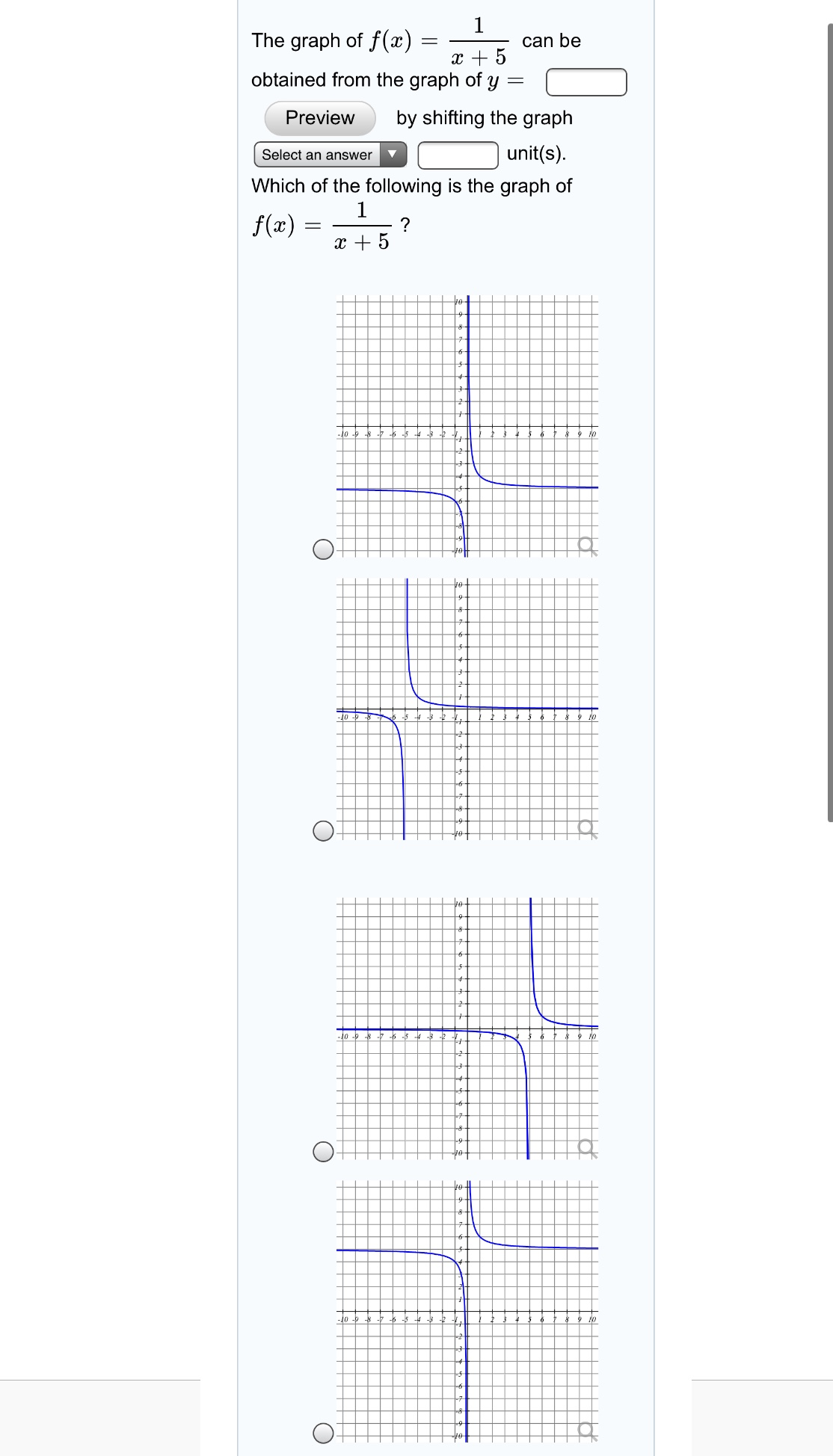# The graph of f(x)can bex + 5obtained from the graph of y =by shifting the graphPreviewunit(s).Select an answerWhich of the following is the graph off(æ)x + 5-10-lo

Question
2 viewshelp_outlineImage TranscriptioncloseThe graph of f(x) can be x + 5 obtained from the graph of y = by shifting the graph Preview unit(s). Select an answer Which of the following is the graph of f(æ) x + 5 -10 -lo fullscreen
check_circle

Step 1

The given function is

Step 2
Step 3

Graph the given function...

### Want to see the full answer?

See Solution

#### Want to see this answer and more?

Solutions are written by subject experts who are available 24/7. Questions are typically answered within 1 hour.*

See Solution
*Response times may vary by subject and question.
Tagged in

### Algebra